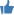## Forum »Achievementsby Naveen Atal (HITWICKETIAN) 81 months ago

## Leading Wicket takers of CKB(13/05/15) T0P 15

NameInningsOversMaidensRunsWicketsAvgEconS.R.Best
2279.1=48750=6.14=14/5
2.HAREENDRA SHARMASHRIMBK XI1664=37639=5.82=22/6
3.CHRIS CARLILETVM Pulikkuttikal1963.4=38845=6.45=14/5
4.SAAHIL SINGHTVM Pulikkuttikal2252.1=33636=6.57=12/4
5.AMIT KHANDELWALuNpLuGgEd UnLeAsHeD
936=19932=5.52=16/6
829.4=12628=4.24=11/6
7.SACHIN AHALEYChampsss
928.1=12728=4.50=10/5
8.NEERAJ SETHIVictory Walkers1232.2=18828=5.81=13/4
1038.4=24028

=

6.20
=19/6
10.HARSHAL KALLATHIL VENRERTVM Pulikkuttikal1948=28027=5.93=25/4
NameInningsOversMaidensRunsWicketsAvgEconS.R.Best
1027=12724=4.70=22/5
12.DEBABRATA KOTOKITVM Pulikkuttikal2262.3=47931=8.03=20/4
825=13521=5.40=19/5
14.RIZWAN AKHTARInvincible Monsters825
11433

13/7
15.DOUGLAS REINHOLDInvincible Monsters
823.3
10821

24/5Views: 893sanjaykarnati (my dream) # 1
CONGO BRO
81 months ago

Refresh posts